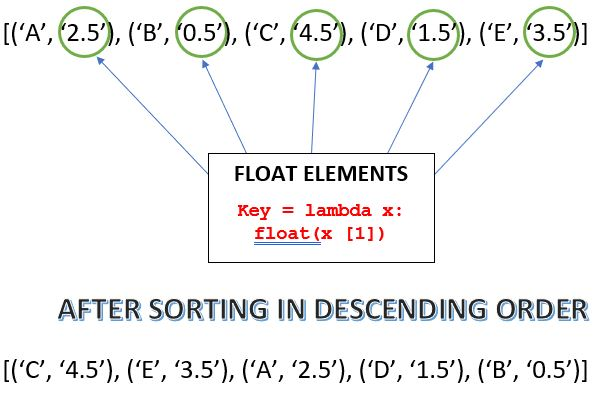# Python | Sort a tuple by its float element

In this article, we will see how we can sort a tuple (consisting of float elements) using its float elements. Here we will see how to do this by using the built-in method sorted() and how can this be done using in place method of sorting.

Examples:

```Input : tuple = [('lucky', '18.265'), ('nikhil', '14.107'),
('akash', '24.541'), ('anand', '4.256'), ('gaurav', '10.365')]
Output : [('akash', '24.541'), ('lucky', '18.265'),
('nikhil', '14.107'), ('gaurav', '10.365'), ('anand', '4.256')]

Input : tuple = [('234', '9.4'), ('543', '16.9'), ('756', '5.5'),
('132', '4.2'), ('342', '7.3')]
Output : [('543', '16.9'), ('234', '9.4'), ('342', '7.3'),
('756', '5.5'), ('132', '4.2')]
```

We can understand this from the image shown below:Method 1 : Use of sorted() method

Sorted() sorts a tuple and always returns a tuple with the elements in a sorted manner, without modifying the original sequence. It takes three parameters from which two are optional, here we tried to use all of the three:

1. Iterable : sequence (list, tuple, string) or collection (dictionary, set, frozenset) or any other iterator that needs to be sorted.
2. Key(optional) : A function that would server as a key or a basis of sort comparison.
3. Reverse(optional) : If set true, then the iterable would be sorted in reverse (descending) order, by default it is set as false.

To sort this in ascending order we could have just ignored the third parameter.

 `# Python code to sort the tuples using float element ` `# Function to sort using sorted() ` `def` `Sort(tup): ` `    ``# reverse = True (Sorts in Descending order) ` `    ``# key is set to sort using float elements ` `    ``# lambda has been used ` `    ``return``(``sorted``(tup, key ``=` `lambda` `x: ``float``(x[``1``]), reverse ``=` `True``)) ` ` `  `# Driver Code ` `tup ``=` `[(``'lucky'``, ``'18.265'``), (``'nikhil'``, ``'14.107'``), (``'akash'``, ``'24.541'``),  ` `    ``(``'anand'``, ``'4.256'``), (``'gaurav'``, ``'10.365'``)] ` `print``(Sort(tup)) `

Output:

```[('akash', '24.541'), ('lucky', '18.265'), ('nikhil', '14.107'),
('gaurav', '10.365'), ('anand', '4.256')]
```

Method 2 : In-place way of sorting using sort():

While sorting via this method the actual content of the tuple is changed, while in the previous method the content of the original tuple remained the same.

 `# Python code to sort the tuples using float element ` `# Inplace way to sort using sort() ` `def` `Sort(tup): ` `    ``# reverse = True (Sorts in Descending order) ` `    ``# key is set to sort using float elements ` `    ``# lambda has been used ` `    ``tup.sort(key ``=` `lambda` `x: ``float``(x[``1``]), reverse ``=` `True``) ` `    ``print``(tup) ` ` `  `# Driver Code ` `tup ``=` `[(``'lucky'``, ``'18.265'``), (``'nikhil'``, ``'14.107'``), (``'akash'``, ``'24.541'``),  ` `    ``(``'anand'``, ``'4.256'``), (``'gaurav'``, ``'10.365'``)] ` `Sort(tup) `

Output:

```[('akash', '24.541'), ('lucky', '18.265'), ('nikhil', '14.107'),
('gaurav', '10.365'), ('anand', '4.256')]
```

For more reference visit:
sorted() in Python
lambda in Python

Whether you're preparing for your first job interview or aiming to upskill in this ever-evolving tech landscape, GeeksforGeeks Courses are your key to success. We provide top-quality content at affordable prices, all geared towards accelerating your growth in a time-bound manner. Join the millions we've already empowered, and we're here to do the same for you. Don't miss out - check it out now!

Previous
Next